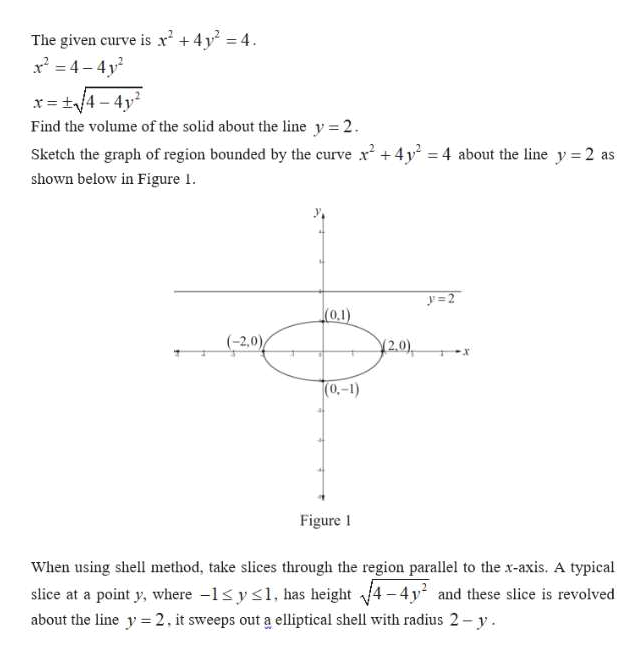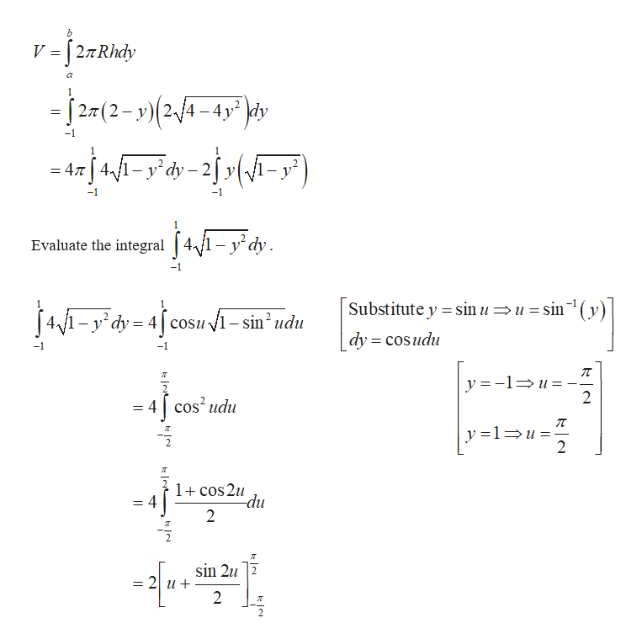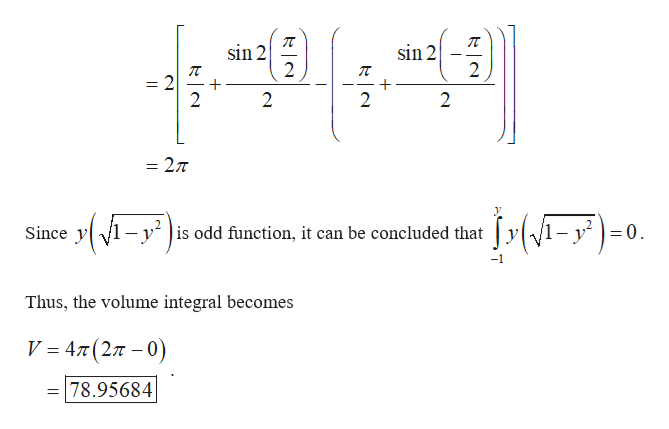Set up an integral for the volume of the solid obtained by rotating the region bounded by the given curves about the specified line. Then use your calculator to evaluate the integral correct to five decimal places.x2 + 4y2 = 4  About y=2About x=2

Question

Set up an integral for the volume of the solid obtained by rotating the region bounded by the given curves about the specified line. Then use your calculator to evaluate the integral correct to five decimal places.

x2 + 4y2 = 4

Step 1

Find the volume of the solid.help_outlineImage TranscriptioncloseThe given curve is r 4y 4 24-4y x=t/4-4y2 Find the volume of the solid about the line y 2 Sketch the graph of region bounded by the curve +4y-4 about the line y 2 as shown below in Figure 1 y=2 (0.1) (-2,0) 2.0) |(0.-1) Figure When using shell method, take slices through the region parallel to the x-axis. A typical slice at a point y, where -s ys, has height about the line y 2. it sweeps out a elliptical shell with radius 2- y V4 -4y and these slice is revolved fullscreen
Step 2

Calculate the volume of the solid about y=2.help_outlineImage TranscriptioncloseV =[27Rhdy -2(2-y)24-4p - rj4-y d=2j »{A=y") -4y dy Evaluate the integral 4/1-y dy -1 Substitute y sinuusin' dy cosudu 41-ydy 4cosui-sin udu = -1 y-1u 2 = 4| cos udu y 1u = 2 1 cos2u du 2 sin 2u 2 u+ 2 fullscreen
Step 3

On further simpli...help_outlineImage Transcriptionclosesin 2 2 sin 2 2 2 2 2 2 = 27T j:(=F)-0. (-y") Since y is odd function, it can be concluded that Thus, the volume integral becomes V 4T(2T -0) 78.95684 fullscreen

Want to see the full answer?

See Solution

Want to see this answer and more?

Our solutions are written by experts, many with advanced degrees, and available 24/7

See Solution
Tagged in

Other